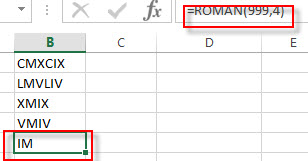# Excel ROMAN Function

This post will guide you how to use Excel ROMAN function with syntax and examples in Microsoft excel.

### Description

The Excel ROMAN function converts an arabic numeral to roman and the format is Text for returned value.

The ROMAN function is a build-in function in Microsoft Excel and it is categorized as a Math and Trigonometry Function.

The ROMAN function is available in Excel 2016, Excel 2013, Excel 2010, Excel 2007, Excel 2011 for Mac.

### Syntax

The syntax of the ROMAN function is as below:

`=ROMAN (number, [form])`

Where the ROMAN function arguments are:

• number -This is a required argument. The arabic numeral that you want to convert.
• Form -This is an optional argument.it will specify the form of the Roman numeral returned. The Roman numeral style ranges from Classic to Simplified, becoming more concise as the value of form increases.
 Form Type 0 or omitted Classic. 1 More concise. See example below. 2 More concise. See example below. 3 More concise. See example below. 4 Simplified. TRUE Classic. FALSE Simplified.

Note:

• If the number is negative, the Function will return #VALUE! Error.
• If the number is greater than 3999, the ROMAN function will return #VALUE! Error.

### Excel ROMAN Function Examples

The below examples will show you how to use Excel ROMAN Function to convert an arabic numeral to roman.

1# to get the classic Roman numeral style for number 999, enter the following formula in Cell B1.

`=ROMAN(999,0)`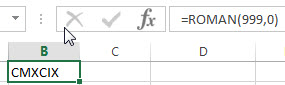2# to get more concise version for number 218, enter the following formula in Cell B2.

`=ROMAN(999,1)`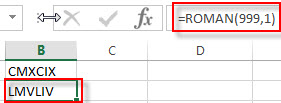3# to get more concise version for number 218 (the Form type is 2), enter the following formula in Cell B3.

`=ROMAN(999,2)`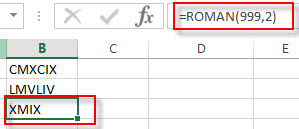4# to get more concise version for number 218 (the Form type is 3), enter the following formula in Cell B4.

`=ROMAN (999,3)`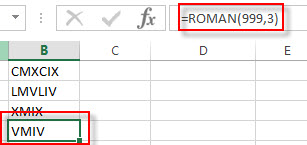5 to get the simplified roman numeral style for number 218 (the Form type is 4), enter the following formula in Cell B5.

`=ROMAN (999,4)`